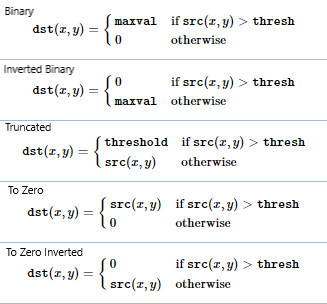# Python | Thresholding techniques using OpenCV | Set-1 (Simple Thresholding)

• Difficulty Level : Easy
• Last Updated : 12 Oct, 2021

Thresholding is a technique in OpenCV, which is the assignment of pixel values in relation to the threshold value provided. In thresholding, each pixel value is compared with the threshold value. If the pixel value is smaller than the threshold, it is set to 0, otherwise, it is set to a maximum value (generally 255). Thresholding is a very popular segmentation technique, used for separating an object considered as a foreground from its background. A threshold is a value which has two regions on its either side i.e. below the threshold or above the threshold.
In Computer Vision, this technique of thresholding is done on grayscale images. So initially, the image has to be converted in grayscale color space.

```If f (x, y) < T
then f (x, y) = 0
else
f (x, y) = 255

where
f (x, y) = Coordinate Pixel Value
T = Threshold Value.```

In OpenCV with Python, the function cv2.threshold is used for thresholding.

Syntax: cv2.threshold(source, thresholdValue, maxVal, thresholdingTechnique)
Parameters:
-> source: Input Image array (must be in Grayscale).
-> thresholdValue: Value of Threshold below and above which pixel values will change accordingly.
-> maxVal: Maximum value that can be assigned to a pixel.
-> thresholdingTechnique: The type of thresholding to be applied.

### Simple Thresholding

The basic Thresholding technique is Binary Thresholding. For every pixel, the same threshold value is applied. If the pixel value is smaller than the threshold, it is set to 0, otherwise, it is set to a maximum value.
The different Simple Thresholding Techniques are:

• cv2.THRESH_BINARY: If pixel intensity is greater than the set threshold, value set to 255, else set to 0 (black).
• cv2.THRESH_BINARY_INV: Inverted or Opposite case of cv2.THRESH_BINARY.
• cv.THRESH_TRUNC: If pixel intensity value is greater than threshold, it is truncated to the threshold. The pixel values are set to be the same as the threshold. All other values remain the same.
• cv.THRESH_TOZERO: Pixel intensity is set to 0, for all the pixels intensity, less than the threshold value.
• cv.THRESH_TOZERO_INV: Inverted or Opposite case of cv2.THRESH_TOZERO.Below is the Python code explaining different Simple Thresholding Techniques –

## Python3

 `# Python program to illustrate``# simple thresholding type on an image``    ` `# organizing imports``import` `cv2``import` `numpy as np` `# path to input image is specified and ``# image is loaded with imread command``image1 ``=` `cv2.imread(``'input1.jpg'``)` `# cv2.cvtColor is applied over the``# image input with applied parameters``# to convert the image in grayscale``img ``=` `cv2.cvtColor(image1, cv2.COLOR_BGR2GRAY)` `# applying different thresholding``# techniques on the input image``# all pixels value above 120 will``# be set to 255``ret, thresh1 ``=` `cv2.threshold(img, ``120``, ``255``, cv2.THRESH_BINARY)``ret, thresh2 ``=` `cv2.threshold(img, ``120``, ``255``, cv2.THRESH_BINARY_INV)``ret, thresh3 ``=` `cv2.threshold(img, ``120``, ``255``, cv2.THRESH_TRUNC)``ret, thresh4 ``=` `cv2.threshold(img, ``120``, ``255``, cv2.THRESH_TOZERO)``ret, thresh5 ``=` `cv2.threshold(img, ``120``, ``255``, cv2.THRESH_TOZERO_INV)` `# the window showing output images``# with the corresponding thresholding``# techniques applied to the input images``cv2.imshow(``'Binary Threshold'``, thresh1)``cv2.imshow(``'Binary Threshold Inverted'``, thresh2)``cv2.imshow(``'Truncated Threshold'``, thresh3)``cv2.imshow(``'Set to 0'``, thresh4)``cv2.imshow(``'Set to 0 Inverted'``, thresh5)``  ` `# De-allocate any associated memory usage ``if` `cv2.waitKey(``0``) & ``0xff` `=``=` `27``:``    ``cv2.destroyAllWindows()`

Input:Output:My Personal Notes arrow_drop_up